## General Chemistry

The Charle’s law shows the correlation between the temperature and the volume of an ideal gas. Let’s discuss it by using a pump with a freely moving plunger that is filled with some gas. In the experiment, we are going to heat up the pump and monitor how the pressure and volume are affected. Remember, we assume that the pressure is constant because the plunger can move freely, and it has no weight.

As expected, the gas is expanding, and we can conclude that the volume of a gas increases with temperature: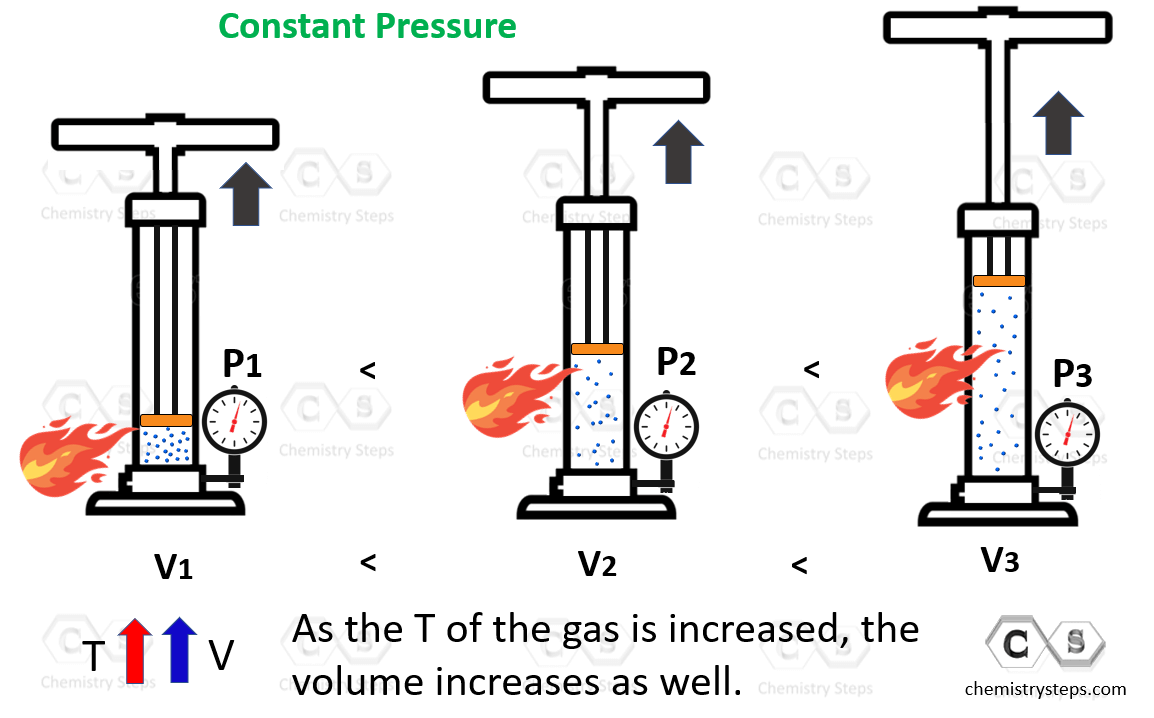The volume is directly proportional to the temperature of the gas, so we can write that: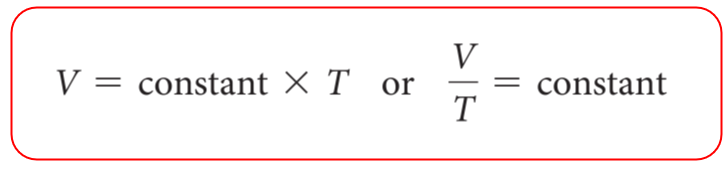$\frac{{\rm{V}}}{{\rm{T}}}{\rm{ = constant}}$

This can be explained using the example of a car dealership income. The income depends on the number of sales which we can represent as:

Income number of cars

However, we cannot say income = number of cars sold, so to switch an equal sign, we need to introduce a constant. This can be the price of the car transforming the equation to:

Income  = price x number of

cars

So, for our experiment, we are not interested too much in the constant, but rather in how it links the temperature and volume of the gas at positions 1 and 2. Because the ratio V/T is constant at any temperature and volume, we can write that:

$\frac{{{{\rm{V}}_1}}}{{{{\rm{T}}_{\rm{1}}}}}\, = \,{\rm{constant}}\, = \,\frac{{{{\rm{V}}_2}}}{{{{\rm{T}}_{\rm{2}}}}}$

or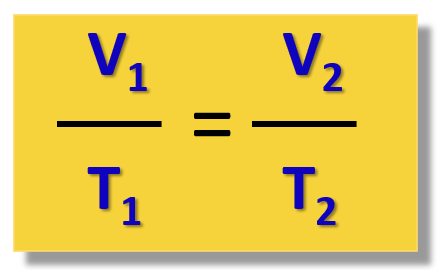This is the practical implication of Charle’s law that is used for solving gas problems.

For example,

What will be the final volume of a 3.50 L sample of nitrogen at 20 °C if it is heated to 200. °C?

Write down what is given and what needs to be determined first:

V1 = 3.50 L

T1 = 20 oC

T2 = 200. oC

V2 = ?

Now, before doing anything else, remember to always convert the temperature to Kelvin when solving a gas problem: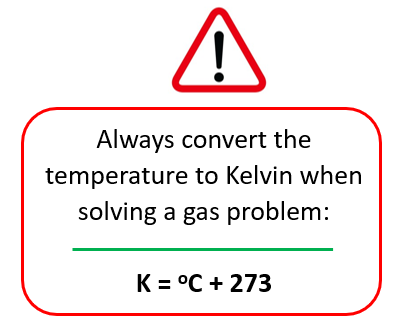So, T1 = 20 + 273 = 293 K,  T2 = 200 + 273 = 473 K

The question studies the correlation between the volume and the temperature of a gas, so we need to use the Charle’s law.

Write it down and rearrange it to get an expression of V2.

$\frac{{{{\rm{V}}_{\rm{1}}}}}{{{{\rm{T}}_{\rm{1}}}}}\; = \;\frac{{{{\rm{V}}_{\rm{2}}}}}{{{{\rm{T}}_{\rm{2}}}}}$

${{\rm{V}}_{\rm{2}}}\; = \;\frac{{{\rm{473}}\;\cancel{{\rm{K}}}\;{\rm{ \times }}\;{\rm{3}}{\rm{.50}}\;{\rm{L}}}}{{{\rm{293}}\;\cancel{{\rm{K}}}}}\;{\rm{ = }}\;{\rm{5}}{\rm{.65}}\;{\rm{L}}$

# The Ideal Gas Law

Notice that in the experiment, we made an assumption that the pressure is constant, and this is what we also do the other gas laws to determine the desired correlation. For example, in the Boyle’s law, we study a constant amount of gas at constant temperature and find that the pressure increase as the volume is decreased.

To combine all the laws together and have the four variables (n, P, V, T) in one place, the Ideal Gas Law equation is obtained: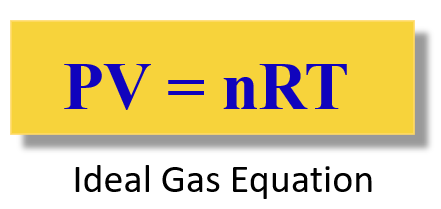The R is called the ideal gas constant. Although it has different values and units, you will mostly be using this:

$R\;{\rm{ = }}\;{\rm{0}}{\rm{.08206}}\;\frac{{{\rm{L}} \cdot {\rm{atm}}}}{{{\rm{mol}} \cdot {\rm{K}}}}$

The ideal gas law equation is used when you need to find P, V, T, or n, for the system where they do not change.

We will cover the ideal gas law in a separate post with some examples.

# How do I know which gas law to use?

You are probably wondering about this question now that every gas law brings a new equation. For this, there is what is called the combined gas law and as long as you remember it, you do not need to remember all the gas laws to solve a problem.

Let’s keep it for another article because there is quite a lot of information in this one.

Check Also